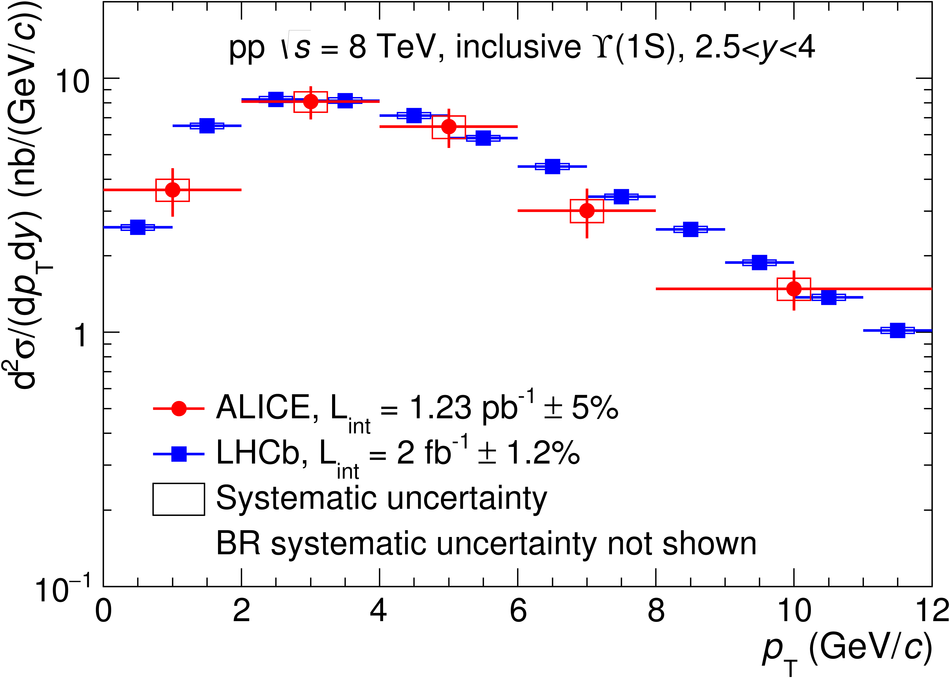# Inclusive quarkonium production at forward rapidity in pp collisions at $\sqrt{s}=8$ TeV

We report on the inclusive production cross sections of J/$\psi$, $\psi$(2S), $\Upsilon$(1S), $\Upsilon$(2S) and $\Upsilon$(3S), measured at forward rapidity with the ALICE detector in pp collisions at a center-of-mass energy $\sqrt{s}=8$ TeV. The analysis is based on data collected at the LHC and corresponds to an integrated luminosity of 1.28 pb$^{-1}$. Quarkonia are reconstructed in the dimuon-decay channel. The differential production cross sections are measured as a function of the transverse momentum $p_{\rm T}$ and rapidity $y$, over the $p_{\rm T}$ ranges $0<~p_{\rm T}<~20$ GeV/$c$ for J/$\psi$, $0<~p_{\rm T}<~12$ GeV/$c$ for all other resonances, and for $2.5<~y<~4$. The cross sections, integrated over $p_{\rm T}$ and $y$, and assuming unpolarized quarkonia, are $\sigma_{{\rm J}/\psi} = 8.98\pm0.04\pm0.82$ $\mu$b, $\sigma_{\psi{\rm (2S)}} = 1.23\pm0.08\pm0.22$ $\mu$b, $\sigma_{\Upsilon{\rm(1S)}} = 71\pm6\pm7$ nb, $\sigma_{\Upsilon{\rm(2S)}} = 26\pm5\pm4$ nb and $\sigma_{\Upsilon{\rm(3S)}} = 9\pm4\pm1$ nb, where the first uncertainty is statistical and the second one is systematic. These values agree, within at most $1.4\sigma$, with measurements performed by the LHCb collaboration in the same rapidity range.

Figures

## Figure 1

 Dimuon invariant mass distributions in the region of charmonia (left) and bottomonia (right). Dashed lines correspond to the background. Solid lines correspond to either the signal functions, or the sum of all signal and background functions. In the charmonia region, the sum of two extended Crystal Ball functions is used for the signal and a pseudo-Gaussian function is used for the background. In the bottomonia region, the sum of three extended Crystal Ball functions is used for the signal and the sum of two exponential functions is used for the background.## Figure 2

 $\jpsi$ (top) and $\psi\prime$ (bottom) differential cross sections as a function of $\pt$ (left) and $y$ (right). $\jpsi$ results are compared to LHCb measurement at $\sqrt{s}$=8 TeV. Open symbols are the reflection of the positive-$y$ measurements with respect to $y=0$. Vertical error bars are the statistical uncertainties. Boxes are the systematic uncertainties. Branching ratio uncertainties are not included.## Figure 3

 Differential cross section of $\Upsilon$(1S) as a function of $\pt$ (left) and differential cross sections of $\Upsilon$(1S), $\Upsilon$(2S) and $\Upsilon$(3S) as a function of $y$ (right) measured by ALICE and LHCb. Open symbols are the reflection of the positive-$y$ measurements with respect to $y=0$.## Figure 4

 $\psi\prime$-to-$\jpsi$ cross section ratio as a function of $\pt$ (left) and $y$ (right).